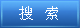说明：双击或选中下面任意单词，将显示该词的音标、读音、翻译等；选中中文或多个词，将显示翻译。 您的位置：首页 -> 句库 -> 递归公式 1. Computing Acyclic Direct Network Reliability Based on First Depth Search一个计算无圈有向网络可靠性的递归公式 2. A Recurrence Formula of ζ(2t) (t is a positive integer);Riemann Zeta函数ξ(2t)(t为正整数)的一个递归公式 3. In this paper, using recurssion formaula of the adjoint polynomial, we shall obtain adjoint polynomials of two graphs. 本文运用伴随多项式的递归公式，求出了两类图的伴随多项式。 4. The Recursive Equation of Homogeneous Linear Recurrent Sequence of Number Formed by General Term Formula; 由通项公式构造齐次线性递归数列的递归方程 5. The General Formula of A Usual Type of Linear Recursive Sequence;一般形式的线性递归数列的通项公式 6. Method for matrix solution of constant coefficient recursive sequences general term equation; 常系数递归数列通项公式的矩阵求法 7. Infinite recursion detected during execution of calculated cell formula %1 at pass %2.在传递 %2 上计算单元公式 %1 的执行过程中检测到无限递归。 8. Prove and Application of Sequences General Term Equation;一类线性递归数列通项公式的证明和应用 9. Methods for obtaining the general term formulae of a kind of non-linear、non-homogeneous recursive sequences; 一类非线性、非齐次递归数列的通项公式的求法 10. Initial Value Problem of Binary Linear Recurrence Relation and Calculation Formula of Second-order Matrix Square Power; 二元线性递归关系的初值问题与二阶矩阵方幂的计算公式 11. Change of Variables for General Formula of Non-homogeneous Linear Recursive Sequence with Constant Coefficients; 常系数非齐次线性递归数列通项公式计算的通项变换法 12. The Methods of Mathematical Thought in Finding the General Term of First Order Constant Coefficient Linear Recursive Sequence; 求一阶常系数线性递归数列通项公式的数学思想方法 13. The paper introduces the general form of recursive model, the process of recursive program running and the recu-rsive program design. 介绍了递归模型的一般形式、归程序的执行过程及递归程序设计。 14. The Recursive and Non-recursive Backtrack Algorithms for Nine Different Numerals Constitute an Equation of Multiplication 九位不同数字乘法等式的递归与非递归回溯算法 15. One of Distributed Recursive Queries Based on XML基于XML的分布式数据库递归查询优化 16. It mainly investigates a special method for recursive program design and its applications a-bout the complicated recursion from the angle of the mathematical formular. 重点从数学公式的角度探讨了复杂递归问题的一类特殊编程方法及其应用。 17. The Recursion Relization of the Greatest Common Divisor of Many Numbers with the Continuous Minus Method; 辗转相减法求多个数的最大公约数的递归实现 18. Cannot call constructor recursively (directly or indirectly)无法以直接或间接方式递归调用构造函数 ©2011 dictall.com